10.4 Insertion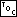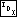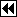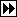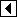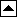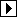Here is the outline:

```377. <RTBST item insertion function 377> =
void **rtbst_probe (struct rtbst_table *tree, void *item) {
struct rtbst_node *p; /* Current node in search. */
int dir;              /* Side of p on which to insert the new node. */

struct rtbst_node *n; /* New node. */

<Step 1: Search RTBST for insertion point 378>
<Step 2: Insert new node into RTBST tree 379>
}
```

This code is included in 375.

The code to search for the insertion point is not unusual:

```378. <Step 1: Search RTBST for insertion point 378> =
if (tree->rtbst_root != NULL)
for (p = tree->rtbst_root; ; p = p->rtbst_link[dir])     {
int cmp = tree->rtbst_compare (item, p->rtbst_data, tree->rtbst_param);
if (cmp == 0)
return &p->rtbst_data;
dir = cmp > 0;

if (dir == 0)         {
if (p->rtbst_link == NULL)
break;
}       else /* dir == 1 */         {
if (p->rtbst_rtag == RTBST_THREAD)
break;
}
}
else   {
p = (struct rtbst_node *) &tree->rtbst_root;
dir = 0;
}
```

This code is included in 377.

Now for the insertion code. An insertion to the left of a node p in a right-threaded tree replaces the left link by the new node n. The new node in turn has a null left child and a right thread pointing back to p: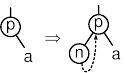An insertion to the right of p replaces the right thread by the new child node n. The new node has a null left child and a right thread that points where p's right thread formerly pointed: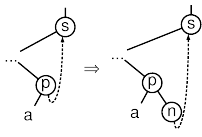We can handle both of these cases in one code segment. The difference is in the treatment of n's right child and p's right tag. Insertion into an empty tree is handled as a special case as well:

```379. <Step 2: Insert new node into RTBST tree 379> =
n = tree->rtbst_alloc->libavl_malloc (tree->rtbst_alloc, sizeof *n);
if (n == NULL)
return NULL;

tree->rtbst_count++;
n->rtbst_data = item;
if (dir == 0)   {
if (tree->rtbst_root != NULL)